﻿ Remap Hue | Sample Dialogs | Imaging Sample Common Dialogs Help
In This Topic ▼

Remap Hue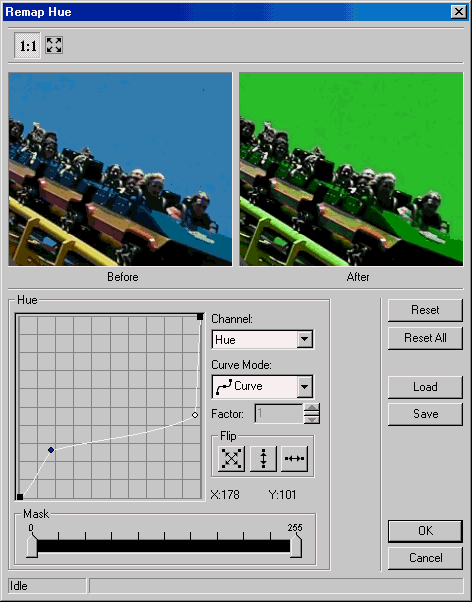This dialog has the following fields:

Channel Drop-down list box:

Use this to select the channel to remap. There are three choices:

• Hue: remaps the Hue channel, for the values within the mask range.

• Saturation: remaps the Saturation channel, for the values within the mask range.

• Value: remaps the Value channel, for the values within the mask range.

Curve Mode Drop-down list box:

Use this to select the curve mode to use when remapping. There are four choices:

•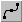Curve: remaps the selected channel using curve data.

•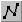Linear: remaps the selected channel using linear data.

•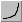Exponential: remaps the selected channel using exponential data, which is affected by the Factor value.

•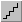Logarithm: remaps the selected channel using logarithmic data, which is affected by the Factor value.

Factor Edit box:

Use this to set the factor of the Exponential/Logarithm curve. Enter the value directly in the edit box or use the arrows to set the desired value.

Flip Buttons:

Use these buttons to flip the curve if the Curve Mode is either Curve or Linear. The following options are available:

•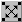Both. Flip the curve vertically and horizontally.

•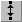Vertically. Flip the curve vertically.

•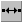Horizontally. Flip the curve horizontally.

Use the Mask Multi-slider Control to specify the range of values for which to apply the changes specified by the channel, the Curve mode and the factor. The first marker from the left is the minimum value of the range, and the second is the maximum value.

Reset Button:

Use the Reset button to set the curve of the current channel to the default values.

Reset All Button:

Use the Reset All button to set the curve for all the channels to default values.

Use the Load button to load an "*.lrh" file that contains Remap Hue curve settings, using the Load dialog. The loaded file will set a new curve for each channel.

✎ NOTE